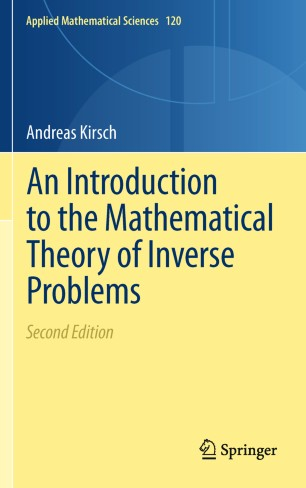# An Introduction to the Mathematical Theory of Inverse Problems

• Andreas KirschTextbook

Part of the Applied Mathematical Sciences book series (AMS, volume 120)

1. Front Matter
Pages i-xiv
2. Andreas Kirsch
Pages 1-21
3. Andreas Kirsch
Pages 23-61
4. Andreas Kirsch
Pages 63-119
5. Andreas Kirsch
Pages 121-165
6. Andreas Kirsch
Pages 167-189
7. Andreas Kirsch
Pages 191-245
8. Back Matter
Pages 247-307

### Introduction

This book introduces the reader to the area of inverse problems. The study of inverse problems is of vital interest to many areas of science and technology such as geophysical exploration, system identification, nondestructive testing and ultrasonic tomography.

The aim of this book is twofold: in the first part, the reader is exposed to the basic notions and difficulties encountered with ill-posed problems. Basic properties of regularization methods for linear ill-posed problems are studied by means of several simple analytical and numerical examples.

The second part of the book presents three special nonlinear inverse problems in detail - the inverse spectral problem, the inverse problem of electrical impedance tomography (EIT), and the inverse scattering problem.

The corresponding direct problems are studied with respect to existence, uniqueness and continuous dependence on parameters. Then some theoretical results as well as numerical procedures for the inverse problems are discussed.

In this new edition, the Factorization Method is included as one of the prominent members in this monograph. Since the Factorization Method is particularly simple for the problem of EIT and this field has attracted a lot of attention during the past decade a chapter on EIT has been added in this monograph.

The book is highly illustrated and contains many exercises. This together with the choice of material and its presentation in the book are new, thus making it particularly suitable for graduate students in mathematics and engineering.

### Keywords

Electrical Impedance Tomography Factorization Method Inverse Problems inverse scattering problem inverse spectral problem

#### Authors and affiliations

• Andreas Kirsch
• 1
1. 1.Department of MathematicsKarlsruhe Institute of Technology (KIT)KarlsruheGermany